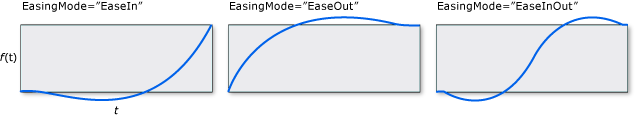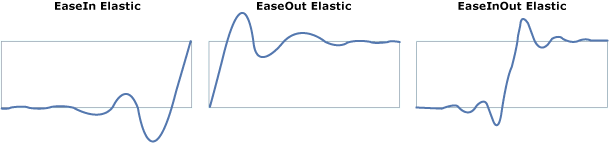# Easing Functions

Easing functions allow you to apply custom mathematical formulas to your animations. For example, you may want an object to realistically bounce or behave as though it were on a spring. You could use Key-Frame or even From/To/By animations to approximate these effects but it would take a significant amount of work and the animation would be less accurate than using a mathematical formula.

Besides creating your own custom easing function by inheriting from EasingFunctionBase, you can use one of several easing functions provided by the runtime to create common effects.

• BackEase: Retracts the motion of an animation slightly before it begins to animate in the path indicated.

• BounceEase: Creates a bouncing effect.

• CircleEase: Creates an animation that accelerates and/or decelerates using a circular function.

• CubicEase: Creates an animation that accelerates and/or decelerates using the formula f(t) = t3.

• ElasticEase: Creates an animation that resembles a spring oscillating back and forth until it comes to rest.

• ExponentialEase: Creates an animation that accelerates and/or decelerates using an exponential formula.

• PowerEase: Creates an animation that accelerates and/or decelerates using the formula f(t) = tp where p is equal to the Power property.

• QuadraticEase: Creates an animation that accelerates and/or decelerates using the formula f(t) = t2.

• QuarticEase: Creates an animation that accelerates and/or decelerates using the formula f(t) = t4.

• QuinticEase: Create an animation that accelerates and/or decelerates using the formula f(t) = t5.

• SineEase: Creates an animation that accelerates and/or decelerates using a sine formula.

To apply an easing function to an animation, use the `EasingFunction` property of the animation specify the easing function to apply to the animation. The following example applies a BounceEase easing function to a DoubleAnimation to create a bouncing effect.

``````<Rectangle Name="myRectangle" Width="200" Height="30" Fill="Blue">
<Rectangle.Triggers>
<EventTrigger RoutedEvent="Rectangle.MouseDown">
<BeginStoryboard>
<Storyboard>
<Storyboard x:Name="myStoryboard">
<DoubleAnimation From="30" To="200" Duration="00:00:3"
Storyboard.TargetName="myRectangle"
Storyboard.TargetProperty="Height">
<DoubleAnimation.EasingFunction>
<BounceEase Bounces="2" EasingMode="EaseOut"
Bounciness="2" />
</DoubleAnimation.EasingFunction>
</DoubleAnimation>
</Storyboard>
</Storyboard>
</BeginStoryboard>
</EventTrigger>
</Rectangle.Triggers>
</Rectangle>
``````

In the previous example, the easing function was applied to a From/To/By animation. You can also apply these easing functions to Key-Frame animations. The following example shows how to use key frames with easing functions associated with them to create an animation of a rectangle that contracts upward, slows down, then expands downward (as though falling) and then bounces to a stop.

``````<Rectangle Name="myRectangle" Width="200" Height="200" Fill="Blue">
<Rectangle.Triggers>
<EventTrigger RoutedEvent="Rectangle.MouseDown">
<BeginStoryboard>
<Storyboard>
<DoubleAnimationUsingKeyFrames
Storyboard.TargetProperty="Height"
Storyboard.TargetName="myRectangle">

<!-- This keyframe animates the ellipse up to the crest
where it slows down and stops. -->
<EasingDoubleKeyFrame Value="30" KeyTime="00:00:02">
<EasingDoubleKeyFrame.EasingFunction>
<CubicEase EasingMode="EaseOut"/>
</EasingDoubleKeyFrame.EasingFunction>
</EasingDoubleKeyFrame>

<!-- This keyframe animates the ellipse back down and makes
it bounce. -->
<EasingDoubleKeyFrame Value="200" KeyTime="00:00:06">
<EasingDoubleKeyFrame.EasingFunction>
<BounceEase Bounces="5" EasingMode="EaseOut"/>
</EasingDoubleKeyFrame.EasingFunction>
</EasingDoubleKeyFrame>

</DoubleAnimationUsingKeyFrames>
</Storyboard>
</BeginStoryboard>
</EventTrigger>
</Rectangle.Triggers>

</Rectangle>
``````

You can use the EasingMode property to alter how the easing function behaves, that is, change how the animation interpolates. There are three possible values you can give for EasingMode:

• EaseIn: Interpolation follows the mathematical formula associated with the easing function.

• EaseOut: Interpolation follows 100% interpolation minus the output of the formula associated with the easing function.

• EaseInOut: Interpolation uses EaseIn for the first half of the animation and EaseOut for the second half.

The graphs below demonstrate the different values of EasingMode where f(x) represents the animation progress and t represents time.

BackEaseBounceEaseCircleEaseCubicEaseElasticEaseExponentialEasePowerEaseQuarticEaseQuinticEaseSineEaseNote

You can use PowerEase to create the same behavior as CubicEase, QuadraticEase, QuarticEase, and QuinticEase by using the Power property. For example, if you want to use PowerEase to substitute for CubicEase, specify a Power value of 3.

In addition to using the easing functions included in the run-time, you can create your own custom easing functions by inheriting from EasingFunctionBase. The following example demonstrates how to create a simple custom easing function. You can add your own mathematical logic for how the easing function behaves by overriding the EaseInCore method.

``````namespace CustomEasingFunction
{
public class CustomSeventhPowerEasingFunction : EasingFunctionBase
{
public CustomSeventhPowerEasingFunction()
: base()
{
}

// Specify your own logic for the easing function by overriding
// the EaseInCore method. Note that this logic applies to the "EaseIn"
// mode of interpolation.
protected override double EaseInCore(double normalizedTime)
{
// applies the formula of time to the seventh power.
return Math.Pow(normalizedTime, 7);
}

// Typical implementation of CreateInstanceCore
protected override Freezable CreateInstanceCore()
{

return new CustomSeventhPowerEasingFunction();
}
}
}
``````
``````Namespace CustomEasingFunction
Public Class CustomSeventhPowerEasingFunction
Inherits EasingFunctionBase
Public Sub New()
MyBase.New()
End Sub

' Specify your own logic for the easing function by overriding
' the EaseInCore method. Note that this logic applies to the "EaseIn"
' mode of interpolation.
Protected Overrides Function EaseInCore(ByVal normalizedTime As Double) As Double
' applies the formula of time to the seventh power.
Return Math.Pow(normalizedTime, 7)
End Function

' Typical implementation of CreateInstanceCore
Protected Overrides Function CreateInstanceCore() As Freezable

Return New CustomSeventhPowerEasingFunction()
End Function

End Class
End Namespace
``````
``````<Window x:Class="CustomEasingFunction.Window1"
xmlns:CustomEase="clr-namespace:CustomEasingFunction"
xmlns="http://schemas.microsoft.com/winfx/2006/xaml/presentation"
xmlns:x="http://schemas.microsoft.com/winfx/2006/xaml"
Title="Window1" Height="500" Width="300">
<StackPanel>
<TextBlock Margin="10" TextWrapping="Wrap">Click on the rectangle to start the animation</TextBlock>
<StackPanel x:Name="LayoutRoot" Background="White">

<Rectangle Name="myRectangle" Width="200" Height="30" Fill="Blue">
<Rectangle.Triggers>
<EventTrigger RoutedEvent="Rectangle.MouseDown">
<BeginStoryboard>
<Storyboard>
<DoubleAnimation From="30" To="300" Duration="00:00:3"
Storyboard.TargetName="myRectangle"
Storyboard.TargetProperty="Height">
<DoubleAnimation.EasingFunction>

easing function.-->
<CustomEase:CustomSeventhPowerEasingFunction EasingMode="EaseIn"/>
</DoubleAnimation.EasingFunction>
</DoubleAnimation>
</Storyboard>
</BeginStoryboard>
</EventTrigger>
</Rectangle.Triggers>

</Rectangle>

</StackPanel>
</StackPanel>

</Window>
``````